# Multiplication Worksheets (Basic Facts 0-10)

We have multiplication sheets for timed tests or extra practice, as well as flashcards and games. Most resources on this page cover basic multiplication facts 0-10.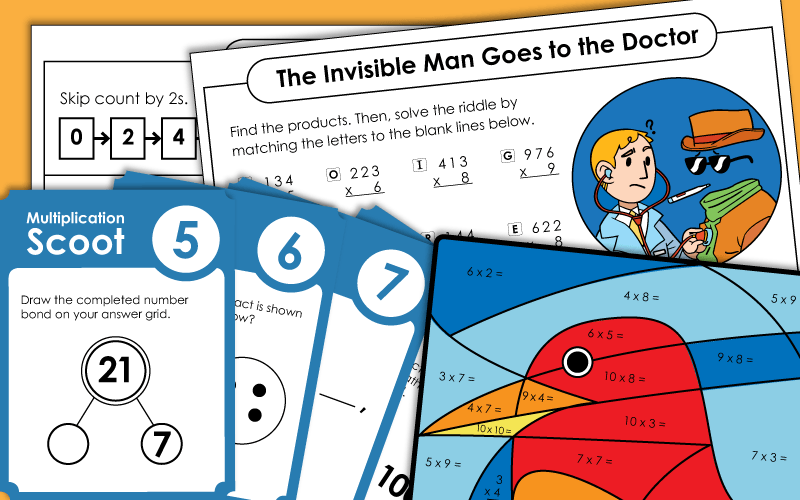## Worksheets andGames

Count the number of groups and the number of objects in each group. This is a 2-page, introductory-level worksheet activity.
Use repeated addition to solve these basic facts. Includes illustrations of fruit as models.
Look closely at each array illustration. Then tell how many columns, how many rows, and how many dots are in each. Then write the corresponding multiplication fact for each array.
Complete the bar models (aka bar diagrams or tape diagrams) and write the multiplication fact for each.
Count the dots on each side of the dominoes and multiply the numbers together.
Write a multiplication and a repeated addition problem for each picture shown.
This PDF contains 30 task cards. Each one has a picture of an array. Students write the multiplication fact shown by each.
Solve the basic multiplication facts on each puzzle piece and match them to the puzzle pieces with their products.
Find the missing factors. Match the problems with the same answers to make stars.
Use the picture clues to help you solve these basic multiplication word problems.
Printable multiplication board game with a space theme.
Cut out a multiplication facts and glue it into the center oval. Then students draw an array, write a repeated addition fact, and create a grouping illustration.
Roll a pair of dice. Multiply the numbers together to find the product.
Each player rolls a pair of dice. They multiply the numbers together to find the product. The player with the largest product wins the round.
Multiply to find out how many ears are on six rabbits, how many legs are on seven spiders, etc.
Try this whole-class, super-fun chain reaction game that teaches basic facts!
Hunt for 20 complete multiplication facts with this printable number search. Circle each fact you find, and write the complete number sentences on the lines provided.
This fun memory card game will help students learn their multiplication facts up to 9 x 9.
30 different multiplication bingo boards and calling cards.
Multiply the number inside the wheel times the number in the middle to find the outside product.
Solve these basic multiplication facts. Then cut out and glue the correct answer to the boxes.
Cut out the tiles at the bottom of the page. Glue the tiles into the boxes to make correct multiplication facts.
In this game, students move from desk to desk, around the classroom, answering basic multiplication questions. Includes 30 game cards, student answer sheet, and teacher instructions.
Unscramble each set of digits to create basic multiplication facts.
These printable multiplication worksheets have a row of multiplication problems all with factors up to 10. Students can write their answers in the space provided.
Fill in the empty squares with factors that will complete the puzzle.
Solve the multiplication facts, then color the boxes according to the key.  Students will find a surprise picture.
These word problems require knowledge of 0 - 9 basic facts.
These linear measurement word problems require only an understanding of basic multiplication facts.
Determine the missing factor for each multiplication fact.
Create number bonds that represent basic multiplication problems.
This origami fortune teller includes the hardest 0-9 multiplication facts. Basic facts include 6x7, 8x7, 6x3, 7x4, 7x3, 3x8, 9x7, and 4x8. (Does NOT include 10s, 11s, or 12s.)
Students write their own rhymes to help learn basic facts.
This is a 2 or 3 player game. Students take turns rolling a die. The number rolled corresponds with a column of math facts on the game board. Students must recite the answers to all facts in the column as quickly as possible.
Use skip counting to learn and practice basic multiplication facts.  Includes facts up to 5 x 12.
More practice with multiplication facts, using skip counting.  Includes basic facts facts up to 10 x 12.
This PDF has 30 multiplication task cards with missing factors. Includes 0-10 multiplication facts.
Write the answers to the multiplication facts, then write X or O over the corresponding numbers on the tic-tac-toe board.
What's a twip? To find out the answer to the funny riddle, answer 33 basic multiplication facts. Then match the products to the letters in the puzzle.
First decode the symbols to reveal the mystery numbers. Then multiply them together and write the product.
A fun crossword puzzle where kids fill in their basic facts.

## Multiplication Tables(Multiplication Charts)

This document contains a completed multiplication table that you can print out.
The products are missing on these mini-multiplication tables. Fill in the products to complete each chart. This worksheet has illustrations of children playing with toy trains and cars.
Write the missing factors and products in the four mini-multiplication tables. This activity has pirate-themed graphics.
THis is an incomplete multiplication. Some numbers are filled in. Students can complete the chart by adding numbers to the empty boxes.
Print 4 miniature multiplication tables on a single page.

## Mystery Pictures

Write the answers to the multiplication facts (0-6) and color according to the key.
Students solve multiplication facts and use the color key to color the picture of a fox.
After solving basic multiplication facts, students color the picture to reveal a fun robot!
This file includes two worksheets. Students will solve basic multiplication problems and color the pages according to their key. The design looks the same, but one worksheet will result in a dog and the other a cat!
Solve the multiplication facts and color to reveal a picture of a rooster. The math facts on this worksheet have factors up to 9.
Will the mystery picture be an apple or a banana? It depends which of the two worksheets your student completes!
Solve the multiplication facts and color to reveal a picture of a bright orange clownfish.
Solve the multiplication facts and color according to the key to reveal a mystery sea monster picture.
This mystery pictures has factors between 0 and 10. The finished picture is a red and orange bird in a nest.
Write the product obtained by multiplying each pair of numbers. Then color the spaces according to the directions at the bottom. The finished picture is a ladybug on a flower.
Solve the multiplication problems and color the page based on your answers. Will you color a police car or fire truck? It depends which of the two worksheets you complete!
Solve the multiplication problems and color according to the key to reveal either an ice cream cone or a summery umbrella.
Will you color in a yummy summer snack or some fun beach toys? It depends which of the two worksheets in this file you complete.

## Basic MultiplicationWorksheet Generator

Basic Multiplication
Worksheet Generator

Make your own basic multiplication worksheets. You choose the range for the first and second factor. This generator allows you to create worksheets with 25 or 50 problems.

## Timed Quizzes

Test students' multiplication skills with this timed quiz with 0s, 1s, and 2s. Facts up to 2x10. Recommended time: 5 minutes.
Here's another timed multiplication test with 0s, 1s, 2s, and 3s.
Time students to see if they can finish this quiz in less than 5 minutes.  Facts include 0s, 1s, 2s, 3s, and 4s.
Try this quick multiplication timed quiz..  Facts include 0s, 1s, 2s, 3s, 4s, and 5s.
This is a five-minute, fifty question multiplication test..  Facts include 0s, 1s, 2s, 3s, 4s, 5s, and 6s.
Build speed and accuracy with this multiplication quiz.  Facts include 0s, 1s, 2s, 3s, 4s, 5s, and 6s.
Here's another quiz for assessing students' knowledge of basic facts.  Facts include 0s, 1s, 2s, 3s, 4s, 5s, 6s, 7s, and, of course, 8s.
When students have learned their facts up to 9x9, try this timed test.  Facts include 0s, 1s, 2s, 3s, 4s, 5s, 6s, 7s, 8s, and 9s.
Yet another timed test for building accuracy and speed.  Facts include 0s, 1s, 2s, 3s, 4s, 5s, 6s, 7s, 8s, 9s, and 10s.

## Multiplication Arrays

Cut out the multiplication facts, array models, and products. Attach each to the correct position on the table.
Use the L-shaped tool to view an array for any multiplication fact up to 10.
Cut out the cards and sorts. Match the facts, products, and array graphics. You can also play a memory match game with these cards.
Teach kids to solve multiplication facts by making arrays with neat rows and columns of symbols.
Students draw their own arrays to solve these multiplication facts.
Kids will love making these creative city skylines using graph paper! This activity helps them practice basic multiplication skills using arrays.

## Fact Families

Use fact family triangles to teach your students about the relationship between multiplication and division.
These multiplication and division problems are shown as a family inside of a graphical house.
These fact families are presented as factor-factor-product groups.

## Flashcards

Copy these flashcards on card stock. Your students can cut them out and practice with them. There's also a sorting sheet on the last page. The facts on these cards are written horizontally. 9 cards per page.
Practice basic multiplication facts with these triangular fact family cards.
Copy these flashcards on card stock and let your students cut them out and practice with them. The facts on these cards are written vertically. 4 cards per page.
These fact families are presented on circles. Students fill in the missing number for each set.

## Properties of Multiplication

According to the distributive property of multiplication, "a multiplication array can be divided into two smaller arrays. When you add the products of the arrays, they equal the product of the larger array."
Here's a slightly more advance worksheet on the distributive property of multiplication.
This is another intermediate-level worksheet that focuses on the distributive property of multiplication.
The commutative property says, "the product of a multiplication problem does not change when you change the order of the numbers."
Learn about the associative property of multiplication, which states that: "the product of a set of numbers is the same, no matter how they are grouped"

More Basic Multiplication

We have thousands of multiplication worksheets. This page will link you to facts up to 12s and fact families. We also have sets of worksheets for multiplying by 3s only, 4s only, 5s only, etc.

(with Multi-Digit Factors)

Fact Families (Basic)

Print basic multiplication and division fact families and number bonds.

Skip Counting Worksheets

Practicing skip counting skills can help students master their multiplication facts.

Properties of Multiplication

Practice using the the distributive, associative, commutative, and identity properties of multiplication.

## Multiplication Worksheet Images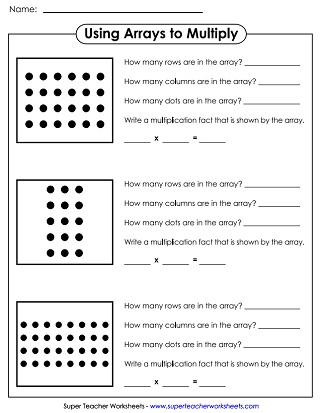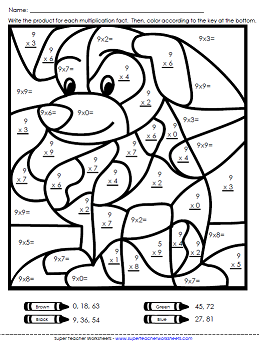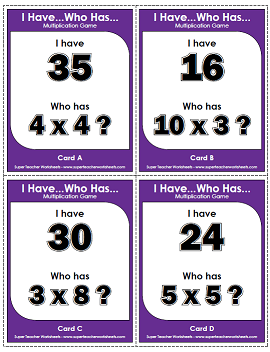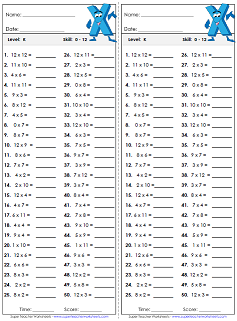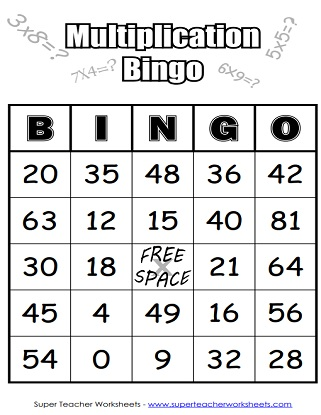My Account
Site Information